# Neighbourhood (topology)

## Contents

In topology, a neighbourhood of a point is any set that belongs to the neighbourhood system at that point. The notion of neighbourhood systems is used to describe, in an abstract setting, the concept of points near a given point, a concept that cannot be expressed by a single set. It is modelled after the situation in real analysis where the points in small balls are considered as near to the centre of the ball.

In a topological space, a set is a neighbourhood of a point if (and only if) it contains the point in its interior, i.e., if it contains an open set that contains the point. However, neighbourhood systems can also be characterized axiomatically and then be used to define the corresponding open sets.

Properties of a point that only depend on conditions restricted to a neighbourhood of the point are often called local properties.

Neighbourhoods are used to define convergence and continuous functions:

Convergence (Definition)
A sequence converges to a point if and only if every neighbourhood of that point contains almost all (i.e., all but finitely many) elements of the sequence.
Continuity (Definition)
A function f is continuous at a point x if and only if for every neighbourhood U of f(x) there is a neighbourhood V of x for which the image f(V) under f is a subset of U.

Neighbourhoods are also used to classify topological spaces according to their separation properties which express how well the points can be distinguished by the topological structure.

## Neighbourhood spaces

A set X is called a neighbourhood space if for every x in X a nonempty family N(x) (the neighbourhood system at x) of sets, called neighbourhoods of x, is given, satisfying the following axioms:

1. x is an element of every neighborhood of x.
2. Any set that contains a neighbourhood of x is a neighbourhood of x.
3. The intersection of any two (and therefore of any finite collection of) neighbourhoods of x is a neighbourhood of x.
4. Any neighbourhood of x contains an open neighbourhood of x,
i.e., a neighbourhood of x that belongs to N(y) for all of its elements y.

Axioms (2-3) imply that N(x) is a filter. Accordingly, the neighbourhood system at a point is also called the neighbourhood filter of the point.
Axiom (4) defines how neighbourhood systems at distinct points interact.

### Neighbourhood base

To define a neighbourhood space it is often more convenient to describe, for all x, only a base for the neighbourhood system.

A nonempty family B(x) of sets is a neighbourhood base at x if it satisfies the following axioms:

1. x is an element of every set in B(x).
2. The intersection of any two sets of B(x) contains a set of B(x).
3. Any set of B(x) contains an open neighbourhood V of x,
i.e., a set V (not necessarily a member of B(x)) that contains some set of B(y) for all of its elements y in V.

Axiom (2) implies that B(x) is a filter base.

(A subbase for the neighbourhood filter is filter subbase, i.e., a family of sets such that its finite intersections form a base for the filter.)

The family N(x) consisting of all sets containing a set of B(x) is the neighbourhood filter induced by B(x).

Two neighbourhood bases B1(x) and B2(x) are called equivalent if they induce the same neighbourhood system N(x) at x. (This is the case if and only if each set in B1(x) contains a set in B2(x), and if, vice versa, each set in B2(x) contains a set in B1(x).)

If there exist countable neighbourhood bases at all x in X, then the corresponding topological (or, equivalently, neighbourhood) space is said to be first-countable.

### Relation to topological spaces

Neighbourhood spaces are one of several equivalent means to define a topological space. The equivalence is obtained by the following definitions:

(Definition)
In a neighbourhood space, a set is open if it is a neighbourhood of all its points.
(Definition)
In a topological space, a set is a neighbourhood of a point if it contains an open set that contains the point. (In other words, the open sets containing a point form a neighbourhood base at this point.)

## Neighbourhood of a set

A set U is called neighbourhood of the set S if U is a neighbourhood of every point of S or, equivalently, if U contains an open set that contains S.

## Examples

### Metric spaces

In a metric space the (open or closed) balls with centre x form a neighbourhood base at x and define the topology induced by the metric.
Moreover, it is sufficient to take the balls with radius 1/n for all natural numbers n (that is, a countable set of balls for each point x), therefore metric spaces are first-countable.

The classical example (in calculus or real analysis) is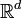$\mathbb R^d$, the d-dimensional Euclidean space:

A neighbourhood base at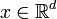$x \in \mathbb R^d$ is given by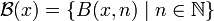$\mathcal B(x) = \left\{ B(x,n) \mid n \in \mathbb N \right\}$
and induces the neighbourhood system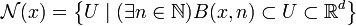$\mathcal N(x) = \left\{ U \mid ( \exists n\in\mathbb N ) B(x,n) \subset U \subset \mathbb R^d \right\}$
where$B(x,n) = \left\{ y \left\vert \left| y-x \right| < {1\over n} \right. , y \in \mathbb R^d \right\} \subset \mathbb R^d$
are the open balls with centre x and radius 1 / n.

While the example assumes the (standard) Euclidean metric, this is not essential. Other common metrics (e.g., derived from the maximum norm or other norms) define neighbourhood bases which are different but equivalent to it and induce the same neighbourhood system.

### Pointwise convergence

For the space of continuous real functions the topology corresponding pointwise convergence can be defined by neighbourhood systems, but not by a metric:

A neighbourhood base at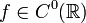$f \in C^0(\mathbb R)$ — the set of continuous real functions — is given by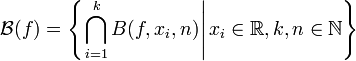$\mathcal B(f) = \left\{\left. \bigcap_{i=1}^k B(f,x_i,n) \right| x_i \in \mathbb R , k,n \in \mathbb N \right\}$
and induces the neighbourhood system$\mathcal N(f) = \left\{ U \left| ( \exists x_i\in\mathbb R )( \exists k,n\in\mathbb N ) \bigcap_{i=1}^k B(f,x_i,n) \subset U \subset C^0(\mathbb R) \right.\right\}$
where the sets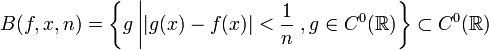$B(f,x,n) = \left\{ g \left\vert \left| g(x)-f(x) \right| < {1\over n} \right. , g \in C^0(\mathbb R) \right\} \subset C^0(\mathbb R)$
form a subbase for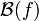$\mathcal B(f)$.Some content on this page may previously have appeared on Citizendium.